# 12 More New Super Useful Tricks In JavaScript12 More New Super Useful Tricks In JavaScript, I collect 12 more techniques that are very practical in front-end or the backend (Node.js) development: Randoms, Encrypt a number, Create Functions Dynamically, Every and Some, Convert to Boolean, Conditionally setting a variable, Swap variables, Shorthand with the && operator, Set mandatory parameters, Beautify JSON programmatically, How to customize with CSS & Template Literals your javascript console, Merging multiple objects

In a previous article, I compile some of the JavaScript “techniques” that I usually use in my development day-to-day. In this new article, I collect 12 more techniques that are very practical in front-end or the backend(nodejs) development, but maybe they are not so well known.

1. Randoms
2. Encrypt a number
3. Create Functions Dynamically
4. Every and Some
5. Convert to Boolean
6. Conditionally setting a variable
7. Swap variables
8. Shorthand with the && operator
9. Set mandatory parameters
10. Beautify JSON programmatically
11. How to customize with CSS & Template Literals your javascript console
12. Merging multiple objects

## 1. Randoms

In the following example, I show two practical ways to generate random numbers or getting a random item from an array.

Generate a random number from a range:

``````let getRandomNumber = (min, max) => {
return Math.round(Math.random() * (max - min) + min);
}
console.log(getRandomNumber(0, 100));``````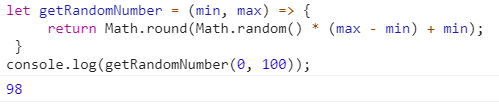getRandomNumber function.

Get a random item from an array:

``````let names= ['Evelyn', 'Harper', 'Jackson', 'Avery', 'Jack', 'Eleanor', 'Carter'];

const chooseName = () =>{
let randomName =
names[Math.floor(Math.random()
*names.length)];
return randomName;
}
console.log('Random name:', chooseName(names));``````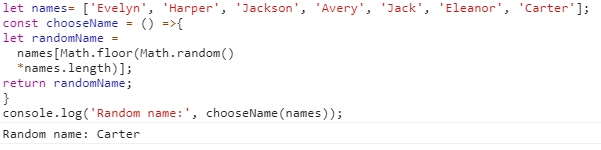chooseName function.

## 2. Encrypt a number

You can use the XOR (^) operator to encrypt and decrypt numbers messages. Here is how it works:

``````const secretKey = 12345;
const plainNumber= "1005"

//Encrypt it:
let encryptedNumber = plainNumber ^ secretKey;
console.log(encryptedNumber);
//Decrypt it:
let decryptedNumber = encryptedNumber ^ secretKey;
console.log(decryptedNumber);``````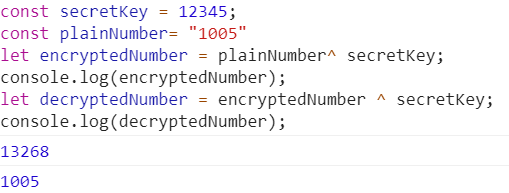Decrypted number.

## 3.Create Functions Dynamically

Create functions dynamically is a useful mechanism to generate functions dynamically based on a string. The first parameter is a comma-separated list of arguments, and the last parameter is the code for the function body:

``````const multiply = new Function("n1", "n2", "return n1 * n2");

console.log(multiply(2, 3));``````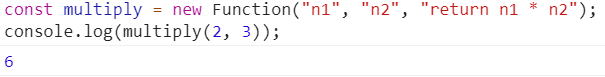Result of the dynamic function.

You can use default parameters (ES6):

``````const multiply = new Function("n1", "n2 = 3", "return n1 * n2");

console.log(multiply(2));``````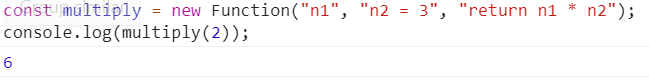Result of the dynamic function.

Or Rest Parameters(ES6) with a reducer(ES6):

``````const multiply = new Function("...numbers", " return numbers.reduce( (a,b) => a * b, 1)" );

console.log(multiply(1,2,3,4));``````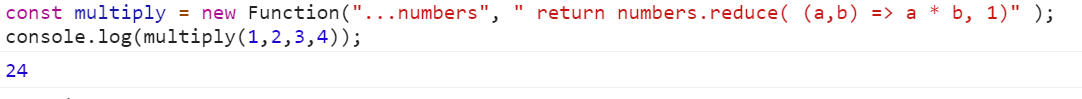Result of the dynamic function.

## 4. Every and Some

The (ES11) Array.every() method in JavaScript is used to check whether all the array elements satisfy the given condition. The Array.some() method in JavaScript, instead, is used to check whether at least one of the array elements satisfies the given condition.

``````const myArray = [10,20,30,9,50]

console.log(myArray.every( e => e > 10));
console.log(myArray.every( e => e > 9));
console.log(myArray.some( e => e > 10));``````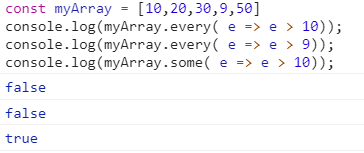Results of the different functions.

## Hire Node.JS Developers | Skenix Infotech

We are providing robust Node.JS Development Services with expert Node.js Developers. Get affordable Node.JS Web Development services from Skenix Infotech.

## A Guide to Web Scraping With JavaScript and Node.js

In this JavaScript Web Scraping tutorial, we’re going to illustrate how to perform web scraping with JavaScript and Node.js. Since JavaScript is excellent at manipulating the DOM (Document Object Model) inside a web browser, creating data extraction scripts in Node.js can be extremely versatile. We’ll start by demonstrating how to use the Axios and Cheerio packages to extract data from a simple website. Then, we’ll show how to use a headless browser, Puppeteer, to retrieve data from a dynamic website that loads content via JavaScript.

## The Ultimate Guide to Web Scraping With JavaScript and Node.js

We’re going to illustrate how to perform web scraping with JavaScript and Node.js. Learn how to use the Axios and Cheerio packages to extract data from a simple website. Learn how to use a headless browser, Puppeteer, to retrieve data from a dynamic website that loads content via JavaScript.